# Overstock of images

» Posted by on Oct 18, 2020 in business, sale

Overstock
Reference Images Size Description
12324×20 Backboard mounted (to keep the print flat)
12530×20 print
12624×20 print
12724×20 print
12820×30 Backboard mounted (to keep the print flat)
12919×23 Print only
130print (24×29) mat (31×26) Mounted with mat
131print (24×20) mat (30×24) Mounted with mat
132print (24×20) mat (30.75 x 26) Mounted with mat
133print (20×24) mat (27.5 x 31.5) Mounted with mat
134print (12×18) frame (25.5×31.5) print + mat
135print (12×18) frame (25.5×31.5) print + mat
136print (12×18) frame (25.5×31.5) print + mat
137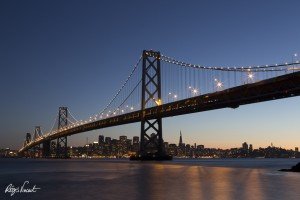print (12×18) frame (25.5×31.5) print + mat
138print (12×18) frame (25.5×31.5) print + mat
139print (12×18) frame (25.5×31.5) print + mat
140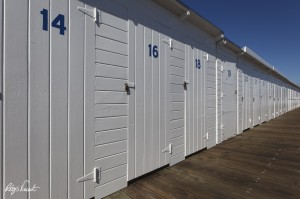print (12×18) frame (25.5×31.5) print + mat
141print (12×18) frame (25.5×31.5) print + mat
144print (12×18) frame (25.5×31.5) print + mat
145print (12×18) frame (25.5×31.5) print + mat
146print (12×18) frame (25.5×31.5) print + mat
49print (12×18) frame (25.5×31.5) print + mat
149print (12×18) frame (25.5×31.5) print + mat
150print (12×18) frame (25.5×31.5) print + mat
151print (12×18) frame (25.5×31.5) print + mat
152print (12×18) frame (25.5×31.5) print + mat
143print (12×18) frame (25.5×31.5) print + mat
148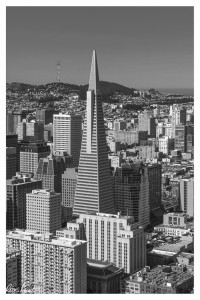print (12×18) frame (25.5×31.5) print + mat
156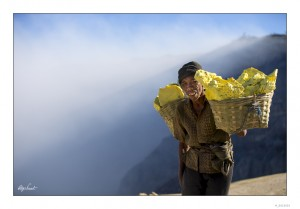print (12×18) frame (25.5×31.5) print + mat
157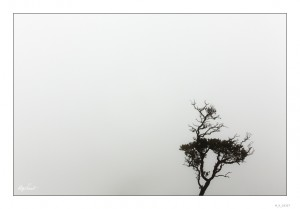print (12×18) frame (25.5×31.5) print + mat
158print (12×18) frame (25.5×31.5) print + mat
159print (12×18) frame (25.5×31.5) print + mat
160print (12×18) frame (25.5×31.5) print + mat